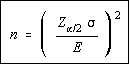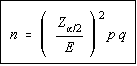Sample Size Determination

The sample size determination formulas come from the formulas for the maximum error of the estimates. The formula is solved for n. Be sure to round the answer obtained up to the next whole number, not off to the nearest whole number. If you round off, then you will exceed your maximum error of the estimate in some cases. By rounding up, you will have a smaller maximum error of the estimate than allowed, but this is better than having a larger one than desired.

Population MeanHere is the formula for the sample size which is obtained by solving the maximum error of the estimate formula for the population mean for n. Again, be sure to round this value up to the nearest whole number.

Population ProportionHere is the formula for the sample size which is obtained by solving the maximum error of the estimate formula for the population proportion for n. Some texts use p hat and q hat, but since the sample hasn't been taken, there is no value for the sample proportion. p and q are taken from a previous study, if one is available. If there is no previous study or estimate available, then use 0.5 for p and q, as these are the values which will give the largest sample size, and it is better to have too large of a sample size and come under the maximum error of the estimate than to have too small of a sample size and exceed the maximum error of the estimate.